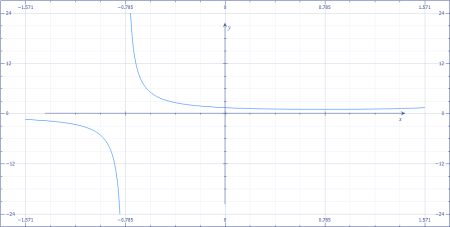# Graph y= \csc(x + \pi/4)on the interval (-\pi/2, \pi/2).

## Question:

Graph {eq}y= \csc(x + \pi/4) {/eq} on the interval {eq}(-\pi/2, \pi/2) {/eq}.

## Graphing

We can graph the given function in various ways. Here, a graphing utility has been used. A specific interval is given and this means that the input variable is limited and we to plot accordingly.

The graph has been plotted below.